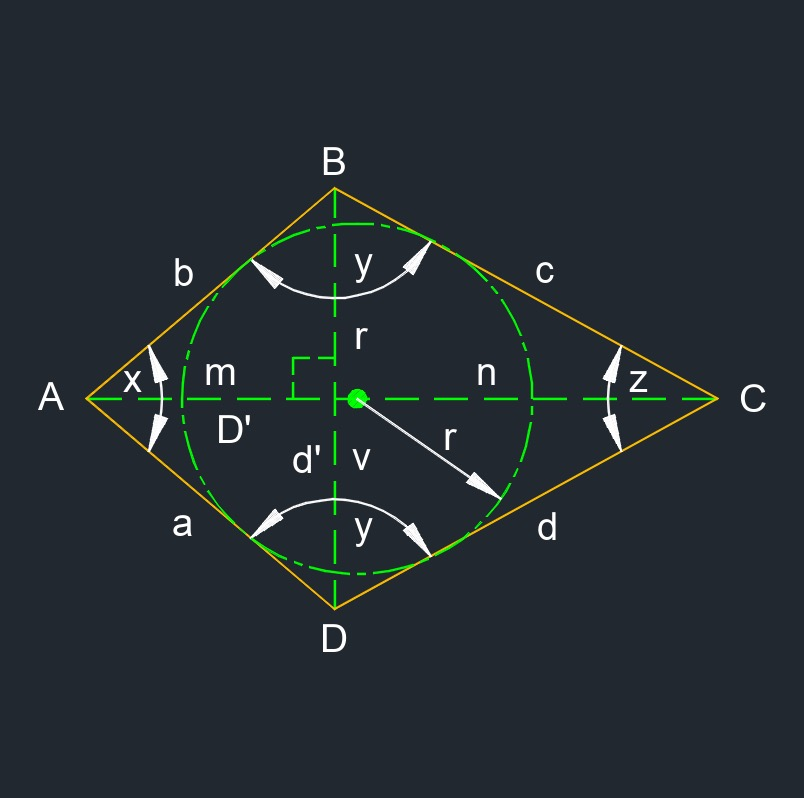# Kite

on . Posted in Plane Geometry

•Kite (a two-dimensional figure) is a quadrilateral with two pairs of adjacent sides that are congruent.
• Acute angle measures less than 90°.
• Congruent is all sides having the same lengths and angles measure the same.
• Diagonal is a line from one vertices to another that is non adjacent.
• Obtuse angle measures more than 90°.
• a = b
• c = d
• ∠B = ∠D
• ∠A ≠ ∠C
• ∠A + ∠B + ∠C + ∠D = 360°
• 2 diagonals
• 4 sides
• 4 vertexs

## Angle of a Kite formulas

$$\large{ x = arccos \; \frac{ m^2 \;+\; a^2 \;-\; \left( \frac{d'}{2} \right)^2 }{ 2\;m\;a } }$$

$$\large{ y = \frac{360° \;-\; x \;-\; z}{2} }$$

$$\large{ z = arccos \; \frac{ \left( D'\;m \; \right)^2 \;+\; d^2 \;-\; \left( \frac{d'}{2} \right)^2 }{ 2\;\left( D'\;m \right)\;d } }$$

Symbol English Metric
$$\large{ x }$$ = acute angle $$\large{ deg }$$ $$\large{ rad }$$
$$\large{ y }$$ = obtuse angle $$\large{ deg }$$ $$\large{ rad }$$
$$\large{ z }$$ = acute angle $$\large{ deg }$$ $$\large{ rad }$$
$$\large{ d' }$$ = diagonal $$\large{ in }$$ $$\large{ mm }$$
$$\large{ D' }$$ = diagonal $$\large{ in }$$ $$\large{ mm }$$
$$\large{ m }$$ = diagonal section $$\large{ in }$$ $$\large{ mm }$$
$$\large{ a, b, c, d }$$ = edge $$\large{ in }$$ $$\large{ mm }$$

## Area of a Kite formulas

$$\large{ A_{area} =\frac { d' \;D' }{2} }$$

$$\large{ A_{area} =\frac{1}{2} \; n \; r }$$

Symbol English Metric
$$\large{ A_{area} }$$ = area $$\large{ in^2 }$$ $$\large{ mm^2 }$$
$$\large{ d' }$$ = diagonal $$\large{ in }$$ $$\large{ mm }$$
$$\large{ D' }$$ = diagonal $$\large{ in }$$ $$\large{ mm }$$
$$\large{ m, n, r, v }$$ = diagonal $$\large{ in }$$ $$\large{ mm }$$

## Diagonal of a Kite formulas

$$\large{ d' = 2\; \frac {A} {D'} }$$

$$\large{ D' = 2\; \frac {A} {d'} }$$

Symbol English Metric
$$\large{ d' }$$ = diagonal $$\large{ in }$$ $$\large{ mm }$$
$$\large{ D' }$$ = diagonal $$\large{ in }$$ $$\large{ mm }$$
$$\large{ A_{area} }$$ = area $$\large{ in^2 }$$ $$\large{ mm^2 }$$

## Edge of a Kite formulas

$$\large{ a = \frac {p} {2} - c }$$

$$\large{ c = \frac {p} {2} - a }$$

Symbol English Metric
$$\large{ a, b, c, d }$$ = edge $$\large{ in }$$ $$\large{ mm }$$
$$\large{ p }$$ = perimeter $$\large{ in }$$ $$\large{ mm }$$

## Perimeter of a Kite formulas

$$\large{ p= 2\; \left( a + c \right) }$$

$$\large{ p= 2\;a + 2\;c }$$

Symbol English Metric
$$\large{ p }$$ = perimeter $$\large{ in }$$ $$\large{ mm }$$
$$\large{ a, b, c, d }$$ = edge $$\large{ in }$$ $$\large{ mm }$$